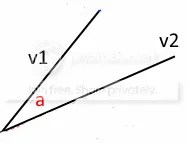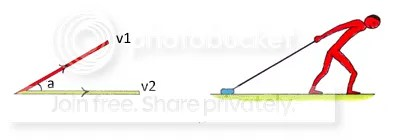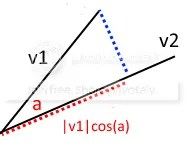You may have seen dot products being used in code, and wondered what they are, and what they are used for. That’s what this post is about.

Let me get the definition out of the way

The dot product of two vectors

1. v1 = (x1, y1) and
2. v2 = (x2, y2)

is written as v1 . v2 and is calculated as

`v1 . v2 = x1*x2 + y1*y2`

which is the same as

`v1 . v2 = |v1||v2|cos(a)`

where

• |v1| is the length of v1 = sqrt(x1*x1 + y1*y1), and |v2| is similar
• a is the angle between v1 and v2 (see diagram below)I have spent a lot of time looking for a clear explanation of what the dot product means. I found plenty of explanations, but nothing very clear. I wasn’t the only one looking – a teacher was also trying to find an understandable meaning for his class. The answer he got was that

we don’t set out to find the dot product. We set out to find angles between vectors, the component of a vector in some direction, the distance of a point from a line or plane, the equation of a plane, and so on and so on, and we use dot products in getting the answers to these questions.

In other words, the dot product is just a convenient mathematical tool.

I’m sure it is, but I’d still like to understand it.

The best explanation I found was this (and I borrowed his picture).

Suppose you are dragging a block along the ground as shown below.The block is moving left to right along the yellow line, but the direction the man is pulling, is at an angle a. So some of his energy is wasted pulling upwards instead of sideways.

Vectors (see previous post) have direction and length (ie size), and

• the energy being put into pulling is the length of the red vector = |v1|
• the distance the block travels is the length of the yellow vector = |v2|
• the total effort put into pulling is energy x distance = |v1||v2|, but some of it is wasted
• the bigger the angle between the two vectors, the more energy is wasted

So how much energy is wasted, and how much is felt by the block?

The geometric answer is to drop a (blue) line from the end of v1, onto v2 at a right angle, as shown below, and calculate the length of the dotted red line. We know that cos(a) = length of dotted red line / |v1| (using trigonometry), so the length of the red line = |v1|cos(a).This is called the “projection of v1 on v2”, and tells us how much of v1 is in the same direction as v2. Going back to our example of the man pulling the block,

1. the length of v1 tells us how hard he is pulling
2. the length of the red line tells us how much of that effort is felt by the block

You can see that if the man pulls sideways in the same direction as the block, so the angle a is zero, then cos(a)=1, and the effort felt by the block is |v1|, which is the full pulling effort.

However, if our man were to pull at an angle of 90 degrees, ie straight up, then cos(90)=0, and the block feels nothing, as we would expect.

My way of understanding all this is that

• cos(a) is the % efficiency of the pulling, so
• if our man pulls with energy of |v1| at an angle of a degrees,
• the actual energy felt by the block is |v1|cos(a), and
• if the block moves a distance of |v2|, total energy felt is |v1||v2|cos(a), or v1 . v2

So, if

1. the effort |v1| = 10, and
2. the distance travelled |v2| = 5,
3. and the angle a = 25 degrees,

then

• the total effort put into pulling the block a distance of 5 = effort x distance = 10 * 5 = 50
• the efficiency of the pulling is cos(25) =0.9 = 90%
• the total effort felt by the block in moving a distance of 5 = v1 . v2 = 10 * 5 * cos(25) = 50 * 0.9 = 45

Question – what if v1 and v2 are reversed? The answer is the same.

There are a number of uses for the dot product

• it tells us how much of v1 is in the same direction as v2
• if it is 0, then v1 is at right angles to v2
• if it is positive, then the angle is less than 90 degrees, and v1 and v2 are pointing in the same general direction
• it gives us an easy way to calculate the angle a (which is really useful if you have 3D vectors, where angle calculations can be difficult)
• it is used in lighting
• it helps in finding the distance of a point from a plane or from a line
• and the shortest distance between two lines in space
• and the equation of a plane defined by three points
• and there are various physics and astronomical and engineering uses